PS.需要 OFFICE 365 才支持！LET 函数是干啥的呢？官方给出的解释是这样的。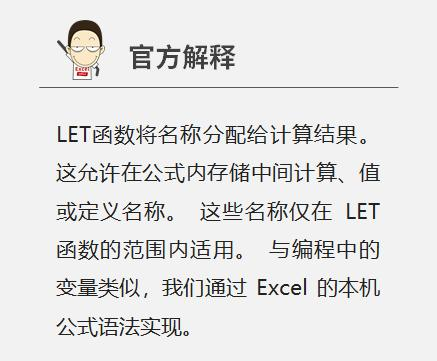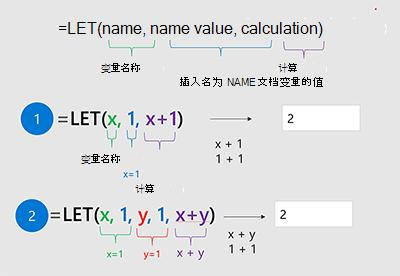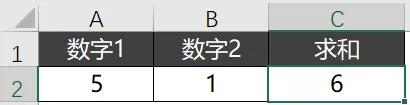`=LET(x,5,SUM(x,1))`

`=LET(x,5,SUM(x,1))`

### 第一步，把 5 保存在 X 中。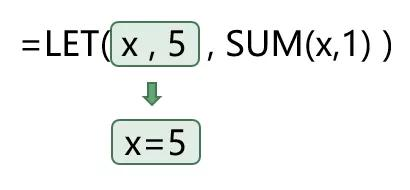### 第二步，用 5 替换写，进行 SUM 计算。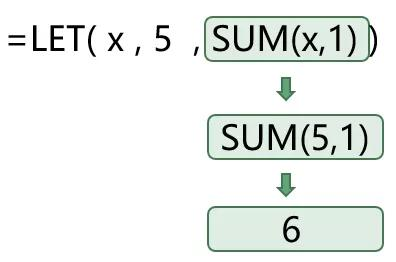### 02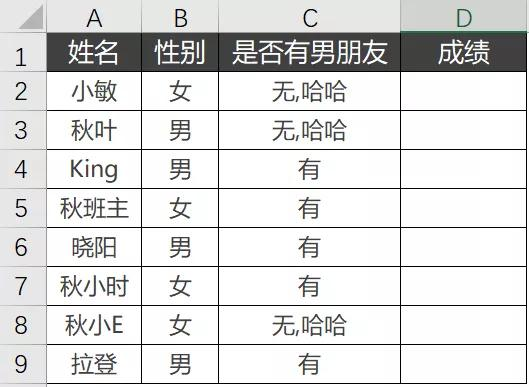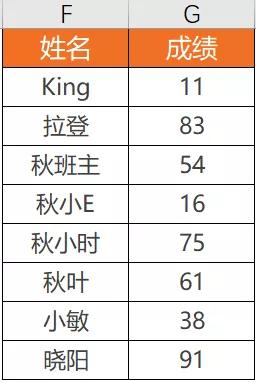❶ 大于等于 60 分的备注及格；

❷ 小于 60 分的显示原有的数字。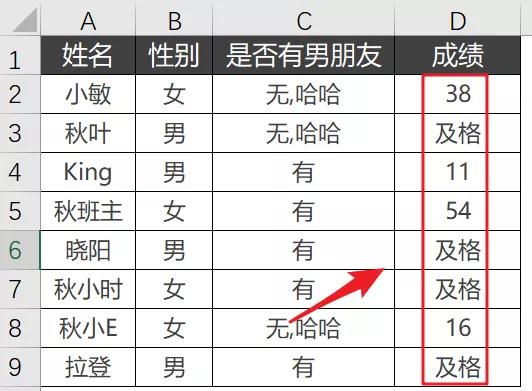`=IF(VLOOKUP(A2,\$F\$1:\$G\$9,2,0)>60,"及格",VLOOKUP(A2,\$F\$1:\$G\$9,2,0))`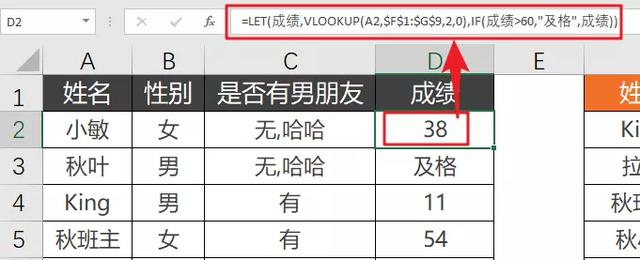`=LET(成绩,VLOOKUP(A2,\$F\$1:\$G\$9,2,0),IF(成绩>60,"及格",成绩))`

### 03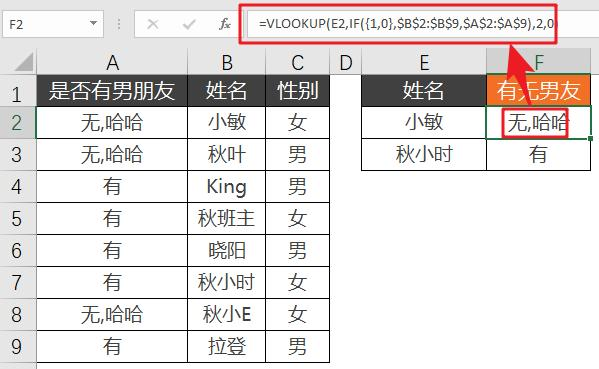`=VLOOKUP(E2,IF({1,0},\$B\$2:\$B\$9,\$A\$2:\$A\$9),2,0)`

`=LET(姓名,\$B\$2:\$B\$9,男友,\$A\$2:\$A\$9,换顺序,IF({1,0},姓名,男友),VLOOKUP(E2,换顺序,2,0))`

❶ 姓名=\$B\$2:\$B\$9；

❷ 男友=\$A\$2:\$A\$9；

❸ 换顺序=IF({1,0},姓名,男友)。### 04

LET 函数好用当然好用，但是还是要注意一些使用规则。

❶ 名称不能是数字；

❷ 名称里不能有运算符

❸ 定义的名称，只能在当前公式中使用。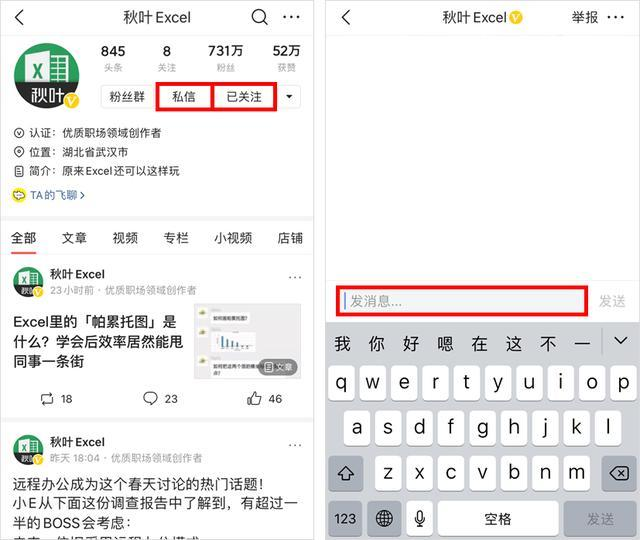想要从零系统学习Excel，查看本站上方“视频教程”获取最新秋叶Office三合一办公应用课程！